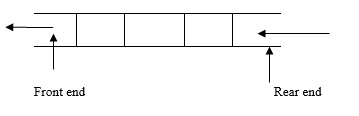# What are the inserting elements in queue in C language?

Data structure is collection of data organized in a structured way. It is divided into two types as explained below −

• Linear data structure − Data is organized in a linear fashion. For example, arrays, structures, stacks, queues, linked lists.

• Nonlinear data structure − Data is organized in a hierarchical way. For example, Trees, graphs, sets, tables.

## Queue

It is a linear data structure, where the insertion is done at rear end and the deletion is done at the front end.The order of queue is FIFO – First In First Out

### Operations

• Insert – Inserting an element into a queue.
• Delete – Deleting an element from the queue.

### Conditions

• Queue over flow − Trying to insert an element into a full queue.

• Queue under flow − Trying to delete an element from an empty queue.

## Algorithms

Given below is an algorithm for insertion ( )

• Check for queue overflow.
if (r==n)
printf ("Queue overflow")
• Otherwise, insert an element in to the queue.
q[r] = item
r++

## Program

Following is the C program for inserting the elements in queue −

#include <stdio.h>
#define MAX 50
void insert();
int array[MAX];
int rear = - 1;
int front = - 1;
main(){
int choice;
while (1){
printf("1.Insert element to queue ");
printf("2.Display elements of queue ");
printf("3.Quit ");
scanf("%d", &choice);
switch (choice){
case 1:
insert();
break;
case 2:
display();
break;
case 3:
exit(1);
default:
printf("Wrong choice ");
}
}
}
void insert(){
if (rear == MAX - 1)
printf("Queue Overflow ");
else{
if (front == - 1)
/*If queue is initially empty */
front = 0;
printf("Inset the element in queue : ");
rear = rear + 1;
}
}
void display(){
int i;
if (front == - 1)
printf("Queue is empty ");
else{
printf("Queue is : ");
for (i = front; i <= rear; i++)
printf("%d ", array[i]);
printf("");
}
}

## Output

When the above program is executed, it produces the following result −

1.Insert element to queue
2.Display elements of queue
3.Quit
Inset the element in queue: 34
1.Insert element to queue
2.Display elements of queue
3.Quit
Enter your choice: 3